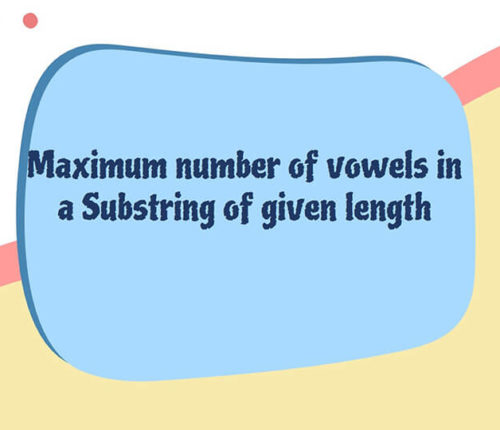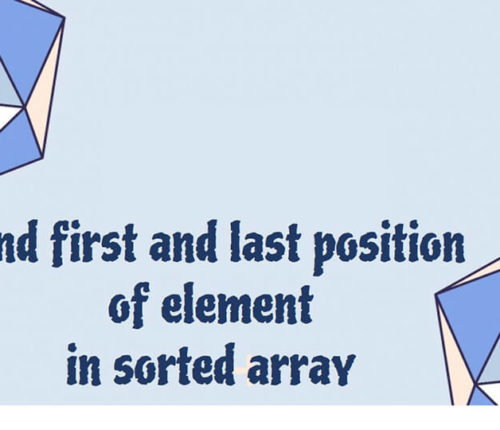# Find subarrays with given sum in an array.

If you want to practice data structure and algorithm programs, you can go through Java coding interview questions.

In this post, we will see how to find subarrays with given sum in an array.

## Problem

Given an Array of non negative Integers and a number. You need to print all the starting and ending indices of Subarrays having their sum equal to the given integer.
For Example :-

Input-int[] arr = {2, 3, 6, 4, 9, 0, 11};
int num = 9
Output-

starting index : 1, Ending index : 2
starting index : 5, Ending index : 5
starting index : 5, Ending index : 6

Explanation :
[3, 6] ,
[9,0] These all are the subarrays with their sum equal to 9.

## Solution

Naive Method:
The basic brute force approach to this problem would be generating all the subarrays of the given array, then loop through the generated subarray and calculate the sum and if this sum is equal to the given sum then printing this subarray as it is the part of our solution.

Now we know, An Array with n elements has n*(n+1)/2 subarrays.
HOW?
Consider an array,

int[] arr = {a1, a2, a3, a4…, an};

Subarrays starting with 0th index,
a1
a1, a2
a1, a2, a3
a1, a2, a3, a4
.
.
.
a1, a2, a3, a4…, an

Subarrays starting with 1st index,
a2
a2, a3
a2, a3, a4
.
.
.
a2, a3, a4…, an

subarrays starting with 2nd index,
a3
a3, a4
.
.
.
a3, a4…, an

Subarrays starting with last i.e. 3rd index,
a4
.
.
.
a4…, an

Now,
Total subarrays = subarrays starting with 0th idx + subarrays starting with 1st idx +
subarrays starting with 2nd idx + . . . + subarrays starting with nth idx

`Sn = n + (n-1) + (n-2) + (n-3) + ... + 1`

`Sn = n(n+1)/2`

There, generating all the subarrays and calculating the answer will cost us the worst time complexity of `O(n(n+1)/2)` which is of the order `O(n^2)`.

Efficient Approach:

We can solve this problem in linear time i.e. in `O(n)` as the worst time complexity.

We can maintain two pointers, `start` and `end` pointers which basically represents a subarray and also we have to take a variable which stores the `current sum` of the subarray starting from start pointer and ending at end pointer.

•  we keep on incrementing `end` pointer while adding the element in the `current sum` until we reach a point where our current running sum is more than required target sum, this basically means that the current subarray whose sum we’ve calculated is not the right answer.
• So now we alter our subarray by moving the `start` pointer, that is shortening the subarray and hence the current sum in the hope that we achieve the current sum equal to the required target sum.
• At every point we check if our current sum is equal to target sum or not, if this is the case we print our pointers.
• So basically we are altering the subarray by increasing `start` and `end` pointers and changing the current sum depending on its value as compared to target sum.

When you run above program, you will get below output:

7
9
2 3 6 4 9 0 11
arr[]: { 2 3 6 4 9 0 11 }
starting index : 1, Ending index : 2
starting index : 4, Ending index : 4
starting index : 4, Ending index : 5

Doubts? Edits? Suggestions? Comment below. Happy Learning 🙂

import_contacts

### You may also like:## Related Posts

•18 June

### Maximum Number of Vowels in a Substring of Given Length

Table of ContentsApproach – 1 Generate All Substrings Using substring() MethodApproach – 2 Using Sliding Window Method (Linear Time Solution) In this article, we will look at an interesting problem related to the Strings and [Sliding-Window Algorithm](https://java2blog.com/sliding-window-maximum-java/ “Sliding-Window Algorithm”). The problem is : "Given a String we have to Find the Maximum Number of Vowel […]

•04 June

### Search for a range Leetcode – Find first and last position of element in sorted array

Table of ContentsApproach 1 (Using Linear Search)Approach 2 (Using Modified Binary Search-Optimal) In this article, we will look into an interesting problem asked in Coding Interviews related to Searching Algorithms. The problem is: Given a Sorted Array, we need to find the first and last position of an element in Sorted array. This problem is […]

•30 April

### Convert Postfix to Infix in Java

Learn about how to convert Postfix to Infix in java.

•30 April

### Convert Prefix to Postfix in Java

Learn about how to convert Prefix to Postfix in java.

•16 April

### Data Structures in java

•29 November

### Top 100+ Java coding interview questions

Table of ContentsStringQuestion 1 : How to reverse a String in java? Can you write a program without using any java inbuilt methods?Question 2 : Write a java program to check if two Strings are anagram in java?Question 3 : Write a program to check if String has all unique characters in java?Question 4 : […]

1.naresh says:
2.Kadane says: